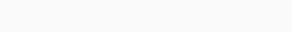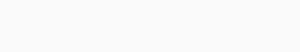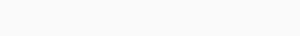# Understanding parallel resistors

When both terminals of a resistor are connected to each terminal of the other resistor or resistors, they are said to be connected in parallel. Unlike series resistor circuits, because there are several channels for current in a parallel resistor network, the circuit current can take multiple paths. Current dividers are then applied to parallel circuits.

In this article, you’ll have an understanding of parallel resistors, their circuit, equation, calculations, diagram, networks, current, and some examples.

Contents

## Resistors in parallel

If the terminals of two resistors are connected to the same two nodes, they are in parallel. The overall equivalent resistance is less than that of the smallest parallel resistor. Because the supply current might travel in many directions, the current may not be the same along all of the parallel network’s branches. In a parallel resistive network, however, the voltage drops across all of the resistors are the same. Then, all parallel linked elements have a common voltage across them, and this is true for all resistors in parallel.

Therefore, a parallel resistive circuit is defined as one in which the resistors are linked to the same two points (or nodes) and is distinguished by the presence of several current paths all coupled to the same voltage source. The voltage across resistor R1 equals the voltage across resistor R2, which equals the voltage across R3, which equals the supply voltage in our parallel resistor example below.

Calculating the equivalent resistance for a resistor in parallel

Considering the diagram below, to form more complex networks, resistors are frequently coupled in series or parallel. In the diagram above, you can see an example of parallel resistors. Each resistor in a parallel circuit has the same voltage across it. The current, on the other hand, is inversely proportional to each resistor’s resistance. The equivalent resistance of many parallel resistors is calculated as follows:

1Req=n∑i=11Ri=1R1+1R2+⋯+1Rn1Req=∑i=1n1Ri=1R1+1R2+⋯+1Rn

Read more: Different types of resistors

The current through each resistor is given by:

Ii=VRiIi=VRi### A quick example of parallel resistance calculation:

A circuit designer must use a resistor with a value of 9 Ω and can select from the E-12 series of preferred values (…, 10, 12, 15, 18, 22, 27, 33, 39, 47, 56, 68, 82, …). The value of 9 Ω is unfortunately not accessible in this series. He chooses to construct an analogous resistance of 9 Ω by connecting two standard values in parallel. These procedures are used to calculate the equivalent resistance value for two parallel resistors:

1Req=1R1+1R21Req=1R1+1R2

1Req=R2R1⋅R2+R1R1⋅R21Req=R2R1·R2+R1R1·R2

1Req=R1+R2R1⋅R21Req=R1+R2R1·R2

Req=R1⋅R2R1+R2Req=R1·R2R1+R2

Read more: Understanding metal oxide film resistors

What the above equation is trying to explain is that, if R1 is equal to R2, then Req is half of the value of one of the two resistors. For a Req of 9 Ω, R1 and R2 should therefore have a value of 2 x 9 = 18 Ω. This happens to be an E-series standard value.

Req=R1⋅R2R1+R2=18⋅1818+18=9ΩReq=R1·R2R1+R2=18·1818+18=9Ω

As a solution, the engineer connects two 18-ohm resistors in parallel to provide an equivalent 9-ohm resistance, as indicated in the diagram below.## Solving a network in parallel and series resistors

The methodical grouping of resistors can typically solve more complex resistor networks. Three resistors are linked as illustrated in the left image below. The resistors R2 and R3 are wired together in series. They are connected in series with resistor R1. The resistors are divided into two groups to solve the network, as shown in the middle image. R1 is the single member of Group 1. R2 and R3 make up Group 2.The sum of R2 and R3 may readily be used to compute the equivalent resistance of group 2:

Rgroup2=R2+R3Rgroup2=R2+R3

This results in the simplified circuit depicted on the right, which has two parallel resistors. This circuit’s equivalent resistance value is simple to calculate:

1Req=1R1+1Rgroup21Req=1R1+1Rgroup2

Req=R1⋅Rgroup2R1+Rgroup2Req=R1·Rgroup2R1+Rgroup2

Req=R1⋅(R2+R3)R1+R2+R3Req=R1·(R2+R3)R1+R2+R3

Larger networks can be solved with repeated use of these principles for calculating parallel and series resistors. Kirchhoff’s laws can also be applied to more complicated networks. The resistors R1, R2, and R3 are all connected in parallel between the two points A and B in the following resistors in a parallel circuit.## Parallel Resistor CircuitThe total resistance of a series resistor network, RT, was equal to the sum of all the individual resistors added together. The equivalent circuit resistance RT is computed differently for parallel resistors.

Instead of adding the resistances directly, the reciprocal (1/R) values of the separate resistances are added together, with the inverse of the algebraic total producing the equivalent resistance as indicated.

## Equation of a Parallel ResistorThe algebraic sum of the inverses of the individual resistances is the inverse of the equivalent resistance of two or more resistors connected in parallel.The total or equivalent resistance, RT, is equal to half the value of one resistor if the two resistances or impedances in parallel are equal and of the same value. R/2, R/3, R/4, R/5, R/6, R/7, R/8, R/9, R/10, R/11, R/12, R/13, R/14, R/15, R/16, R

Because the equivalent resistance is always smaller than the smallest resistor in the parallel network, as more parallel resistors are added, the total resistance, RT, will always drop.

Parallel resistance yields a value known as Conductance, symbol G, with Siemens, sign S, as the unit of conductance. (G = 1/R) Conductance is the reciprocal or inverse of resistance. To convert conductance back to a resistance value, multiply it by the reciprocal, which gives us the total resistance, RT, of the parallel resistors.

We now know that parallel resistor are those that are connected between the same two spots. However, a parallel resistive circuit can take many different shapes besides the one shown above, and below are some examples of how resistors can be linked in parallel.

## Various Resistor Networks in Parallel

Although the five resistive networks below appear to be different, they are all set up as Resistors in Parallel, therefore the same requirements and equations apply.## Resistors in Parallel Calculation Examples

### Example No 1

Take a look at the diagram below. Calculate the total resistance, RT, of the resistors in the following parallel network.The total resistance RT between A and B is calculated as follows:Any number of individual resistances connected together within a single parallel network can be calculated using this reciprocal calculation method.

If there are only two separate resistors in parallel, however, we can determine the total or equivalent resistance value, RT, using a much simpler and faster formula, reducing the reciprocal maths a little.

The following is a considerably faster product-over-sum way of computing two resistors in parallel, with either equal or unequal values:### Resistors in Parallel Example No2

Consider the circuit below, which simply uses two resistors in a parallel configuration.Using the above formula for two resistors connected together in parallel, the total circuit resistance, RT can be calculated as:One thing to keep in mind when using parallel resistors is that the total circuit resistance (RT) of any two resistors connected in parallel is always LESS than the value of the smallest resistor in the combination.

Now let’s look at the current in a parallel resistor circuit which will lead us to the final example.

This video enlighten you on parallel resistors calculations:

## Currents in a Parallel Resistor Circuit

In a parallel resistive circuit, the total current, IT, is the sum of all the individual currents flowing in all the parallel branches. However, because the resistive value of each branch controls the amount of current flowing within that branch, the amount of current flowing through each parallel branch may not be the same.

Although the parallel combination has the same voltage across it, the resistances may differ, and thus the current flowing through each resistor will be different, as specified by Ohms Law.

Consider the two resistors connected in the series above. The current flowing through each of the paralleled resistors (IR1 and IR2) is not always the same because it is determined by the resistive value of the resistor. We do know, however, that the current entering the circuit at point A must likewise exit at point B.

According to Kirchhoff’s Current Laws, “the total current leaving a circuit equals the total current entering the circuit — no current is lost.” As a result, the total current flowing in the circuit is:

IT = IR1 + IR2

Using Ohm’s Law, the current flowing through each parallel resistor in Example No2 may be calculated as follows:

The current flowing through resistor R1 is calculated as follows:

IR1 = VS ÷ R1 = 12V ÷ 22kΩ = 0.545mA or 545μA

The current flowing through resistor R2 is calculated as:

IR2 = VS ÷ R2 = 12V ÷ 47kΩ = 0.255mA or 255μA

So, we have a total current IT flowing around the circuit as:

IT = 0.545mA + 0.255mA = 0.8mA or 800μA

This can also be verified directly using Ohm’s Law as:

IT = VS ÷ RT = 12 ÷ 15kΩ = 0.8mA or 800μA (the same)

The following is the equation for computing the total current flowing in a parallel resistor circuit, which is the sum of all individual currents combined together:

Itotal = I1 + I2 + I3 ….. + In

Because the source current splits or divides amongst the numerous parallel branches, parallel resistor networks can also be thought of as “current dividers.” As a result, a parallel resistor circuit with N resistive networks will have N current routes while retaining a common voltage across itself. Parallel resistors can also be swapped out without affecting the total resistance or current in the circuit.

## Resistors in Parallel Example No3

Calculate the individual branch currents and total current drawn from the power source for the following set of resistors linked in a parallel combination using the diagram below.We may use Ohms Law to determine the individual branch current in a parallel circuit because the supply voltage is shared by all the resistors.Read more: Understanding Capacitors in Series

The total circuit current, IT flowing into the parallel resistor combination will then be:This total circuit current figure of 5 amperes can also be calculated and verified by dividing the supply voltage, VS, by the equivalent circuit resistance, RT, of the parallel branch.

### Equivalent circuit resistance:Then the current flowing in the circuit will be: